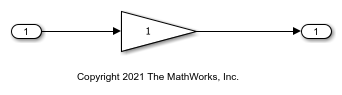Reuse Parameter Data from External Code in the Generated Code

This example shows how to generate code that imports a parameter value from your external code.

Create External Code Files

Suppose your external code defines a vector parameter myGains with three elements. Save the definition in your current folder in a file ex_vector_import_src.c.

#include "ex_vector_import_decs.h"

my_int8 myGains = {
2,
4,
6
};

Save the declaration in your current folder in a file called ex_vector_import_decs.h.

#include "ex_vector_import_cust_types.h"

extern my_int8 myGains;

Save the data type definition my_int8 in your current folder in a file called ex_vector_import_cust_types.h.

typedef signed char my_int8;

Import Parameter Value for Simulation

In your current folder, right-click the file ex_vector_import_src.c and select Import Data.

In the Import dialog box, set Output Type to Numeric Matrix.

Set the name of the generated MATLAB variable to tempVar.

Select only the parameter values (2, 4, and 6) to import.Import the data by clicking the green check mark. The MATLAB variable tempVar appears in the base workspace.

Alternatively, use the command prompt to manually create tempVar.

tempVar = [2;4;6];

At the command prompt, create a Simulink.Parameter object that represents myGains.

Create and Configure Model

Create the model ex_vector_import.

open_system('ex_vector_import')Open the Embedded Coder app.

On the Modeling tab, click Model Data Editor.

In the Model Data Editor, inspect the Parameters tab.

Use the Value column to set the value of the Gain parameter in the Gain block to myGains.

Click the Show/refresh additional information button. The data table now contains a row that represents the parameter object, myGains.

Use the Data Type column to set the data type of myGains to my_int8.

For the other row (which represents the Gain parameter of the Gain block), set Data Type to Inherit: Inherit from 'Gain'. With this setting, the Gain parameter inherits the data type my_int8 from myGains.

Set the Change view drop-down list to Code.

Set these properties for myGains:

• Storage Class to ImportFromFile

Alternatively, use these commands at the command prompt to configure the object and the block:

set_param('ex_vector_import/Gain','Gain','myGains',...
'ParamDataTypeStr','Inherit: Inherit from ''Gain''')
myGains.DataType = 'my_int8';
myGains.CoderInfo.StorageClass = 'Custom';
myGains.CoderInfo.CustomStorageClass = 'ImportFromFile';

At the command prompt, create a Simulink.AliasType object to represent your custom data type my_int8. Set the DataScope and HeaderFile properties to import the type definition from your external code.

my_int8.DataScope = 'Imported';

Set model configuration parameter Source files to ex_vector_import_src.c.

set_param('ex_vector_import','CustomSource','ex_vector_import_src.c')

Generate and Inspect Code

Generate code.

slbuild('ex_vector_import')
### Starting build procedure for: ex_vector_import
### Successful completion of build procedure for: ex_vector_import

Build Summary

Top model targets built:

Model             Action                       Rebuild Reason
=================================================================================================
ex_vector_import  Code generated and compiled  Code generation information file does not exist.

1 of 1 models built (0 models already up to date)
Build duration: 0h 0m 16.712s

The generated file ex_vector_import.h includes the external header files ex_vector_import_decs.h and ex_vector_import_cust_types.h, which contain the parameter variable declaration (myGains) and custom type definition (my_int8).

file = fullfile('ex_vector_import_ert_rtw','ex_vector_import.h');
rtwdemodbtype(file,'#include "ex_vector_import_cust_types.h"',...
'#include "ex_vector_import_cust_types.h"',1,1)
rtwdemodbtype(file,'/* Includes for objects with custom storage classes */',...
'#include "ex_vector_import_decs.h"',1,1)
#include "ex_vector_import_cust_types.h"

/* Includes for objects with custom storage classes */
#include "ex_vector_import_decs.h"

The generated code algorithm in the model step function in the generated file ex_vector_import.c uses myGains for calculations.

file = fullfile('ex_vector_import_ert_rtw','ex_vector_import.c');
rtwdemodbtype(file,'/* Model step function */','/* Model initialize function */',1,0)
/* Model step function */
void ex_vector_import_step(void)
{
/* Outport: '<Root>/Out1' incorporates:
*  Gain: '<Root>/Gain'
*  Inport: '<Root>/In1'
*/
rtY.Out1 = (real_T)myGains * rtU.In1;
rtY.Out1 = (real_T)myGains * rtU.In1;
rtY.Out1 = (real_T)myGains * rtU.In1;
}

The generated code does not define (allocate memory for) or initialize the global variable myGains because the data scope of the corresponding parameter object is imported.

When you simulate the model in Simulink, the model uses the value stored in the Value property of the parameter object. However, if you use external mode simulation, the external executable begins the simulation by using the value from your code. See Considerations for Other Modeling Goals.